# AlphaGo

Posted by lili on

## MiniMax搜索/Alpha-Beta剪枝和象棋

### Alpha-Beta剪枝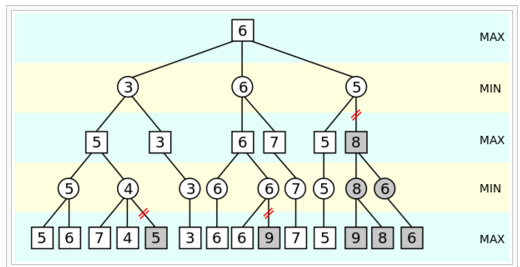图：Alpha-Beta算法图示

alpha-beta能否剪枝非常依赖于搜索的顺序，如果把最优的走法先搜索，那么能得到最大程度的剪枝。所以这个树的展开顺序非常重要。一般会使用很多启发式规则来排序。比如吃对方的棋子很可能是比较好的走法，而没事走动老将不是什么好的走法。

## 围棋为什么不能用类似的方法

### 估值函数

“围棋难的地方在于它的估值函数非常不平滑，差一个子盘面就可能天翻地覆，同时状态空间大，也没有全局的结构。这两点加起来，迫使目前计算机只能用穷举法并且因此进展缓慢。但人能下得好，能在几百个选择中知道哪几个位置值得考虑，说明它的估值函数是有规律的。这些规律远远不是几条简单公式所能概括，但所需的信息量还是要比状态空间本身的数目要少得多（得多）。”

## MCTS介绍

### 多臂老虎机(Multi-Arm Bandits)和UCB(Upper Confidence Bounds)

$R_N=\mu^*n-\sum_{j=1}^{K}\mu_j \mathbb{E}[T_j(n)]$

Lai and Robbins证明了这个损失的下界是logn，也就是说不存在更好的策略，它的损失比logn小（这类似于算法的大O表示法）。所以我们的目标是寻找一种算法，它的损失是log(n)的。UCB就是其中的一种算法：

$UCB1=\bar{X}_j+\sqrt{\frac{2lnn}{n_j}}$

MCTS算法就是从根节点(当前待评估局面)开始不断构建搜索树的过程。具体可以分成4个步骤，如下图所示。

#### Backpropagation

MCTS其实有类似的思想：我们着重搜索更urgent的孩子，所谓urgent，就是更promising的孩子。当然偶尔也要看看那些不那么promising的孩子，说不定是潜力股。这其实就有之前讨论的exploit和explore的平衡的问题。

### UCT算法

UCT算法的公式为：

$UCT=\bar{X}_j+2C_p\sqrt{\frac{2lnn}{n_j}}$

Selection和Expansion的公式如上，和UCB很类似，只是多了一个常量$C_p$，用来平衡exploit和explore。每个节点v都有两个值，N(v)和Q(v)，代表v访问过的次数（每次迭代都是从root到结束状态的一条Path，这条Path上的每个点都被visit一次）和v的回报，如果这次simulation己方获胜，则Q(v)+1，否则Q(v)-1。（1，3，5…层代表自己，2，4，6…代表对手，如果最终我方获胜，1，3，5都要加一而2，4，6都要减一）。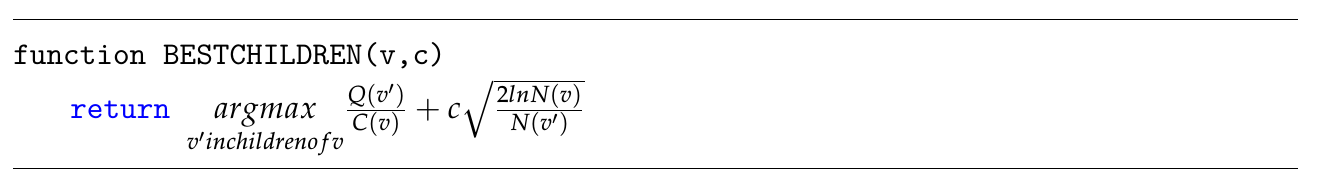• Aheuristic 不需要估值函数，因此也就不需要各种启发式规则，领域知识，从而“回避”了围棋估值函数难的问题。
• Anytime 可以任何时候结束，迭代的次数越多就越准确。
• Asymmetric：前面我们分析过，和人类的搜索类似，搜索树是不对称的，不是固定层次的，而是“重点”搜索promising的子树。

### UCT的变种和改进(可跳过)

AMAF如上图所示，首先通过UCT的Tree Policy我们选择了C2和A1，然后Default Policy我们选择了B1 A3 C3 最终黑棋获胜。

$max\{0, \frac{V-v(n)}{V} \}$

### MCTS的并行搜索

#### Tree Parallelisation

A Lock-free Multithreaded Monte-Carlo Tree Search Algorithm使用了一种lock-free的算法，这种方法比加锁的方法要快很多，AlphaGo也用了这个方法。Segal研究了为什么多机的MCTS算法很难，并且实验得出结论使用virtual loss的多线程版本能比较完美的scale到64个线程（当然这是单机一个进程的多线程程序）。后面我们讨论AlphaGo的scalable的时候会用到这些结论。

## CNN和Move Prediction

### Clark和Storkey的工作图：Maddison等使用的特征

• 第一组feature是棋子（Stone）的颜色，和之前一样。
• 第二组是棋子（所在group）的气，用4个plane来表示，分别是1，2，3 >=4口气。
• 第三组是走了这步棋之后的气，用了6个plane，代表1，2，3，4，5，>=6口气。
• 第四组表示这个走法在当前局面是否合法。
• 第五组表示这个棋子距离当前局面的轮次。 比如上一步对手走的就是1，上上一步自己走的就是2。因为围棋很多都是局部的战役，所以这个feature应该是有用的。
• 第六组就是表示走这这后能吃对方多少个棋子。
• 第八组feature比较有趣，按照作者的说法就是因为KGS的对弈水平参差不齐，如果只留下高手的对局数据太少，所以用这个feature

### Yuandong Tian等人的工作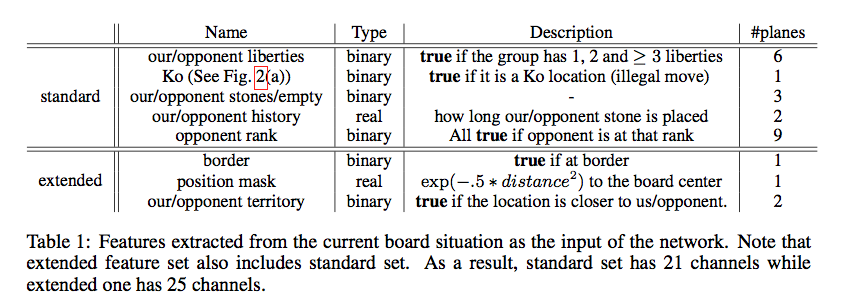图：Yuandong Tian等使用的特征图：Yuandong Tian等的实验结果

1, darkforest: 标准的feature，一步的预测，使用KGS数据

2, darkforest1：扩展的feature，三步预测，使用GoGoD数据

3, darkforest2：基于darkforest1，fine-tuning了一下参数。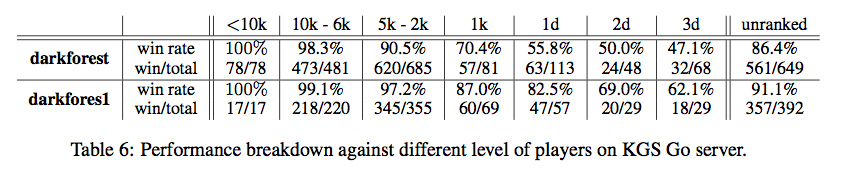图： darkforest的棋力

## AlphaGo的基本原理

### Policy Network和Value Network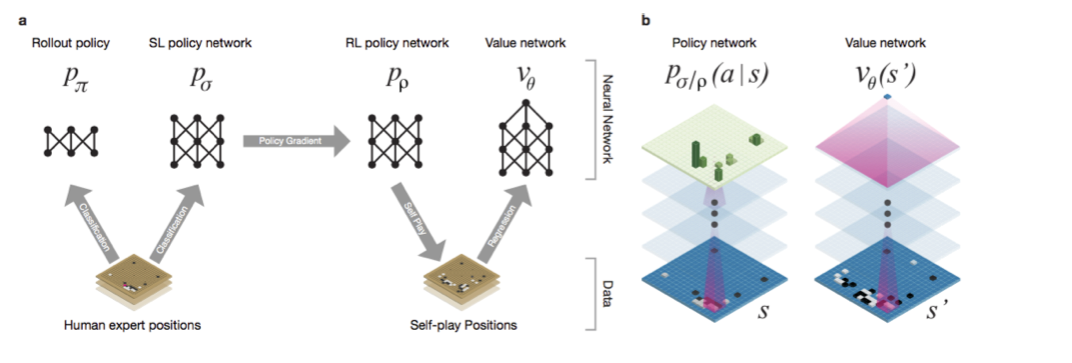图： AlphaGo的Policy Network和Value Network

Policy Network我们通过之前的介绍以及了解到了，它的作用是Tree Policy时候的Node Selection。（rollout阶段不能使用Policy Network，因为DCNN的计算速度相对于Simulation来说太慢，所以AlphaGo又训练了一个简单的Rollout Policy，它基于一些local的pattern之类的feature训练了一个线性的softmax）。

### RL Policy Network和Value Network的训练

$\Delta \rho \propto \frac{\partial logp_{\rho}(a_t|s_t)}{\partial \rho} z_t$

RL Policy Network的初始参数就是SL Policy Network的参数。最后学到的RL Policy Network与SL Policy Network对弈，胜率超过80%。另外RL Policy Network与开源的Pachi对弈（这个能到2d也就是业余两段的水平），Pachi每步做100,000次Simulation，RL Policy Network的胜率超过85%，这说明不用搜索只用Move Prediction能超过2d的水平。这和Tian等人的工作的结论是一致的，他们的darkforest2也只用Move Prediction在KGS上也能到3d的水平。

### Value Network的强化学习训练

$v^p(s)=\mathbb{E}[z_t|s_t=s,a_{t...T} \sim p]$

V(s)的输入时一个局面，输出是一个局面的好坏得分，这是一个回归问题。AlphaGo使用了和Policy Network相同的参数，不过输出是一个值而不是361个值（用softmax归一化成概率）。

$\Delta \theta \propto \frac{\partial v_{\theta}(s)}{\partial \theta}(z-v_{\theta}(s))$

### MCTS + Policy和Value Networks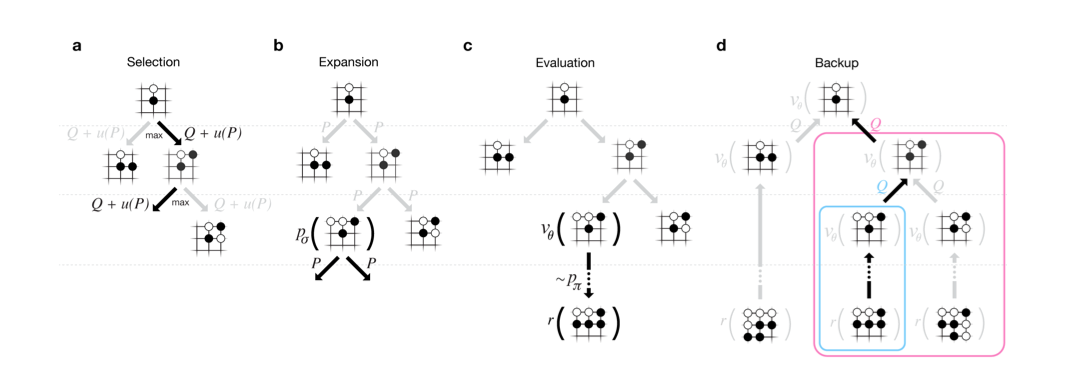图： AlphaGo的MCTS Simulation

#### Selection

$a_t=\underset{a}{argmax}(Q(s_t,a)+u(s_t,a))$

$Q(s,a)$就是exploit term，而$u(s,a) \propto \frac{P(s,a)}{1+N(s,a)}$就是explore term，而且是与先验概率$P(s,a)$相关的，优先探索SL Policy Network认为好的走法。后面的细节部分会介绍$u(s,a)$的详细公式。

#### Evaluation

$V(s_L)=(1-\lambda)v_{\theta}(s_L)+\lambda z_L$

#### Backup

$\begin{split} N(s,a)=\sum_{i=1}^{n}\mathbb{1}(s,a,i) \\ Q(s,a)=\frac{1}{N(s,a)}\sum_{i=1}^{n}\mathbb{1}(s,a,i)V(s_L^i) \end{split}$

n次Simulation之后更新统计量（从而影响Selection），为什么是n次，这涉及到多线程并行搜索以及运行与GPU的Policy Network与Value Network与CPU主搜索线程通信的问题

## AlphaGo的实现细节

### 搜索(Search)算法

$\{ P(s,a),N_v(s,a),N_r(s,a),W_v(s,a),W_r(s,a),Q(s,a) \}$

$P(s,a)$是局面s下走a的先验概率。$W_v(s,a)$是simulation时value network的打分，$W_r(s,a)$是simulation时rollout的打分。$N_v(s,a)$和$N_r(s,a)$分别是simulation时value network和rollout经过边(s,a)的次数。$Q(s,a)$是最终融合了value network打分和rollout打分的最终得分。

rollout会模拟一个节点多次这比较好理解。为什么value network会给同一个节点打分多次呢？而且对于一个DCNN来说，给定一个固定的输入(s,a) $P(a \vert s)$不应该是相同的值吗，计算多次有什么意义吗？

#### Selection(图a)

$\begin{split} a_t &= \underset{a}{argmax}(Q(s_t,a)+u(s_t,a)) \\ u(s,a) &= c_{puct}P(s,a)\frac{\sqrt{\sum_bN_r(s,b)}}{1+N_r(s,a)} \end{split}$

Q(s,a)初始值为0，后面Backup部分会讲怎么更新Q(s,a)。

$u(s_t,a)=c_{puct}P(s,a)\frac{\sqrt{\sum_bN(s,b)}}{1+N(s,a)}$

#### Backup(图d)

$\begin{split} N_r(s_t,a_t) \leftarrow N_r(s_t,a_t)+n_{vl} \\ W_r(s_t,a_t) \leftarrow W_r(s_t,a_t)-n_{vl} \end{split}$

$\begin{split} N_r(s_t,a_t) \leftarrow N_r(s_t,a_t)-n_{vl}+1 \\ W_r(s_t,a_t) \leftarrow W_r(s_t,a_t)+n_{vl}+z_t \end{split}$

$\begin{split} N_v(s_t,a_t) \leftarrow N_v(s_t,a_t)+1 \\ W_v(s_t,a_t) \leftarrow W_v(s_t,a_t)+v_\theta(s_L) \end{split}$

### Distributed APV-MCTS算法

AlphaGo并没有想常见的围棋那样使用AMAF或者RAVE启发，因为这些策略没有什么用处，此外也没有使用开局库，动态贴目(dynamic komi)等。

### Rollout Policy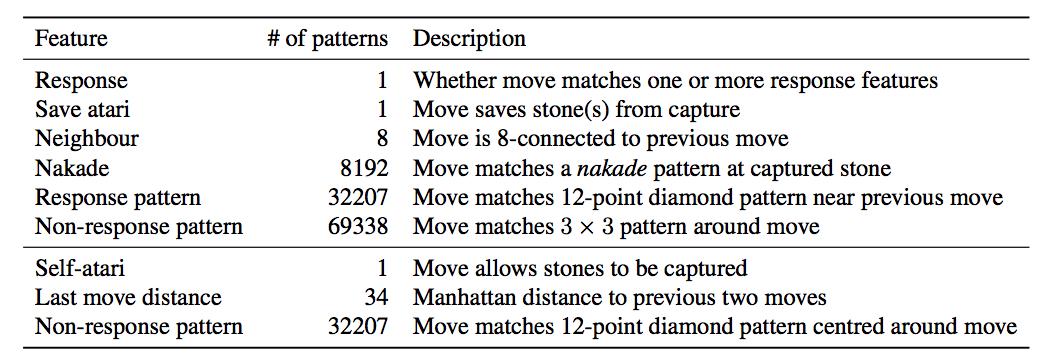图： AlphaGo的Rollout Policy使用的Pattern

### SL Policy Network

SL Policy Network使用了29.4 million局面来训练，这些局面来自KGS 6d-9d 的16万个对局。使用了前1million用来测试，后面的28.4million用来训练。此外进行了旋转和镜像，把一个局面变成8个局面。使用随机梯度下降算法训练，训练的mini-batch大小是16。使用了50个GPU的DistBelief（并没有使用最新的Tensorflow），花了3周的时间来训练了340million次训练步骤(steps)。

### Value Network

• 随机选择一个time-step $U \sim unif\{1,450\}$
• 根据SL Policy Network走1，2，… , U-1步棋
• 然后第U步棋从合法的走法中随机选择
• 然后用RL Policy Network模拟对弈到游戏结束

$s_{U+1},z_{U+1}$被作为一个训练数据加到训练集合里。这个数据是$v^{p_{\rho}}(s_{U+1})-\mathbb{E}[z_{U+1} \vert s_{U+1},a_{U-1,…T} \sum p_{\rho}]$的一个无偏估计。最后这个Value Network使用了50个GPU训练了一周，使用的mini-batch大小是32。

### Policy/Value Network使用的Features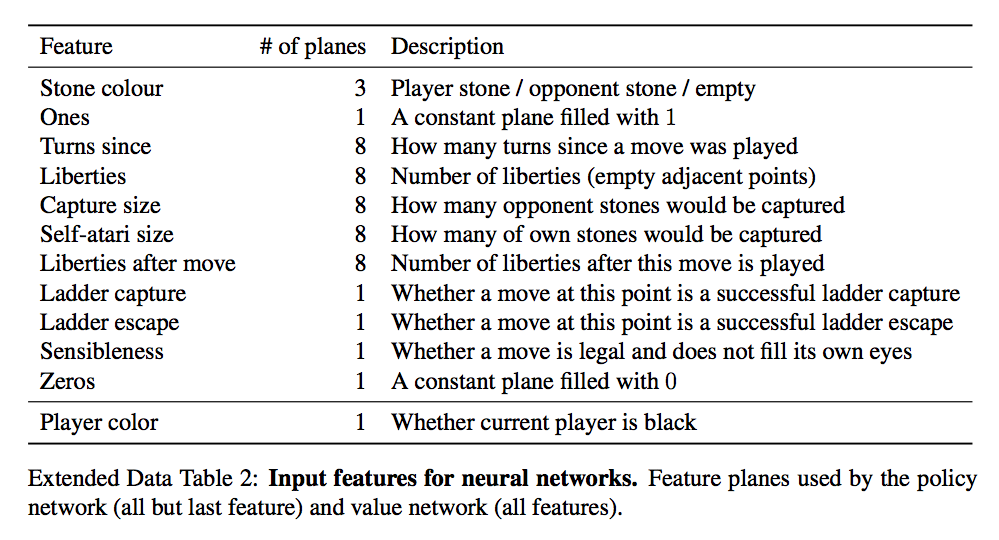图： AlphaGo的Policy/Value Network使用的Features

### 神经网络结构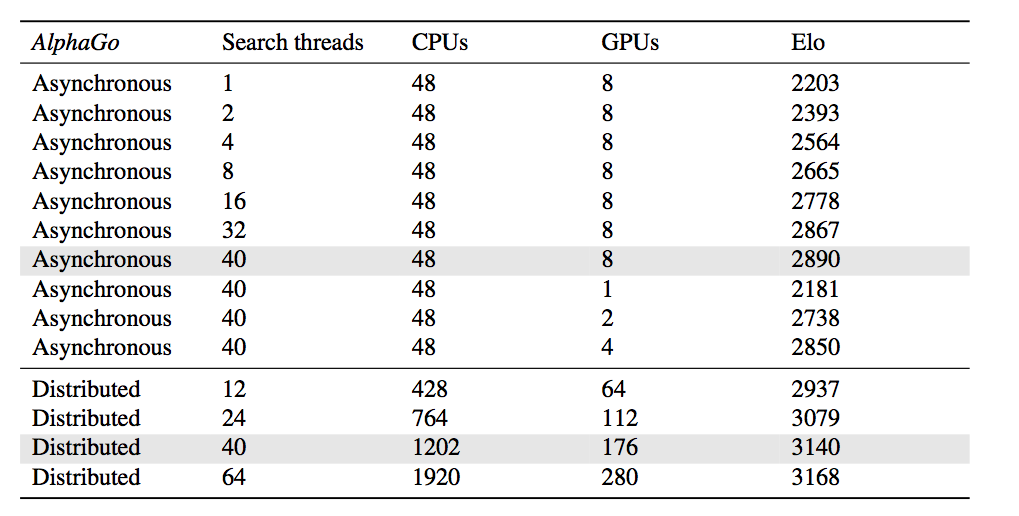图： AlphaGo的Value Network的结构